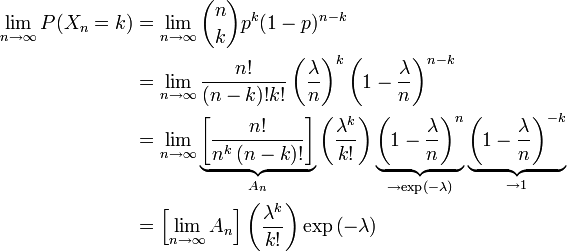# Final-e

Ok, I think I’ve finally got the whole e thing straight in my head.

They key to e, you see, is that it’s arbitrary.

First, my thought progression: one, two and three. Reading over them again, I realize how poorly I understood what was going on because those posts really suck.

Mathematicians are obsessed with two things:

1. Shoving as much information as possible into small spaces; and,
2. Making things look like other things without changing them

As far as I can tell, the history of mathematics is a long string of ‘discoveries’ wherin people learn some kind of identity, like this one that describes e:and feel the faint tickle of recognition. “Hmmm…”, they would say, “now where did I see that before? Ah! Now I remember!” and ka-blammo: apples become oranges that taste like apples.

Think about the calculation of a probability using the Poisson:This is misleadingly complex. The Poisson distribution is NOT complex. The Poisson distribution doesn’t even exist. It’s an approximation of the Binomial distribution, which is freakishly simple:Um… ok, maybe it doesn’t look that simple but that’s because the question it’s designed to answer is a bit complicated: “what is the probability of something happening exactly k times if its probability of happening once is p and you try n times?”

The way of answering it is super easy to understand, though: for one trial the probability is p. For many trials you just start multiplying ys together with (1-p)s, mostly.

Anyway, so what’s the point of the Poisson distribution, then? Well, some clever dude realized this:And what is all that crap. Well that crap is all about the little horizontal curly braces. These are instances where somebody recognized an equation from someplace else and plugged it in. These simplifications remove many steps in calculating the binomial distribution, but increase the difficulty of understanding it.

So e is a massive red herring here. There is no ‘deep truth’ to any of these probability distributions or to the magical math that describes them. You could express a probability using any constant other than e, it’s just that writing it out would be much more complicated and annoying.

Besides, Poisson probabilities are build around the idea of infinite trials. Infinite! There’s no such thing as infinite as far as I’m concerned.

Cute? Yes. Clever? Absolutely. “TRUE” in a deep sense of the word?

Nope.

PS. I was amazed to see what the Binomial distribution is. It’s the effing Normal distribution:No wonder the normal distribution is such a silly concept! It only describes linear, super-simple probabilities. Hmph.